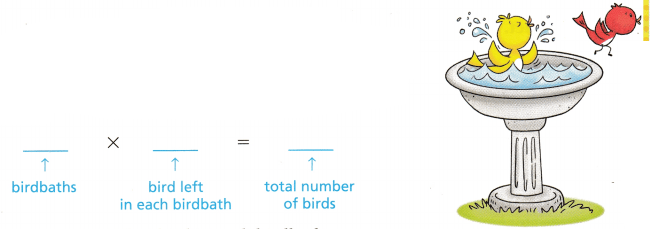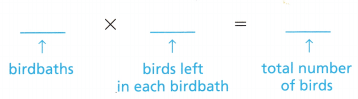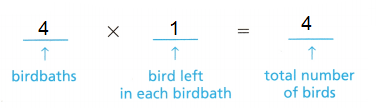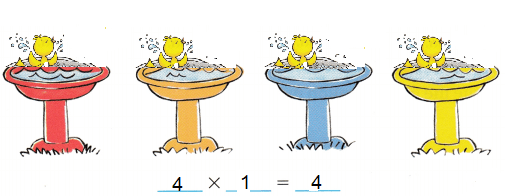# Texas Go Math Grade 3 Lesson 6.6 Answer Key Multiply with 1 and 0

Refer to our Texas Go Math Grade 3 Answer Key Pdf to score good marks in the exams. Test yourself by practicing the problems from Texas Go Math Grade 3 Lesson 6.6 Answer Key Multiply with 1 and 0.

## Texas Go Math Grade 3 Lesson 6.6 Answer Key Multiply with 1 and 0

Essential Question
What happens when we multiply 1 with 0?
0 x 1 = 0
1 x 0 = 0
if we multiply 0 with any number the result will be zero “0”

Unlock the Problem
Luke sees 4 birdbaths. Each birdbath has 2 birds in it. What multiplication sentence tells how many birds there are?

Draw a quick picture to show the birds in the birdbaths.• How many birdbaths are there?
2 x 4 = 8
• How many birds are in each birdbath to start?
2 x 4 = 8

One bird flies away from each birdbath. Cross out 1 bird in each birdbath above. What multiplication sentence
shows the total number of birds left?Now cross out another bird in each birdbath. What multiplication sentence shows the total number of birds left in the birdbaths?• How do the birdbaths look now?Math Talk
Mathematical processes
What if there were 5 birdbaths with 0 birds in each of them? What would be the product? Explain.
5 x 0 = 0
Explanation:
The Zero Property of Multiplication states that the product of zero and any number is zero.

Connect You have multiplied with 1 and 0. Now you will learn two properties that will help you find products when multiplying a number by 1 or by 0.

The Identity Property of Multiplication states that the product of any number and 1 is that number.
7 × 1 = 7 6 × 1 = 6
1 × 7 = 7 1 × 6 = 6
The Zero Property of Multiplication states that the product of zero and any number is zero.
0 × 5 = 0 0 × 8 = 0
5 × 0 = 0 8 × 0 = 0

Share and Show

Question 1.
Find the product. Write the multiplication property you used.
1 × 3 = ___ ___ Property of Multiplication
Identity property
Explanation:
The Identity Property of Multiplication states that the product of any number and 1 is that number.

Find the product.

Question 2.
5 × 1 = ___
Explanation:
The Identity Property of Multiplication states that the product of any number and 1 is that number.

Question 3.
0 × 2 = ___
Explanation:
The Zero Property of Multiplication states that the product of zero and any number is zero.

4 × 0 = ____
Explanation:
The Zero Property of Multiplication states that the product of zero and any number is zero.

Question 5.
1 × 6 = ___
Explanation:
The Identity Property of Multiplication states that the product of any number and 1 is that number.

Question 6.
3 × 0 = ___
Explanation:
The Zero Property of Multiplication states that the product of zero and any number is zero.

Question 7.
1 × 7 = ___
Explanation:
The Identity Property of Multiplication states that the product of any number and 1 is that number.

Question 8.
0 × 0 = ___
Explanation:
The Zero Property of Multiplication states that the product of zero and any number is zero.

Question 9.
8 ×1 = ___
Explanation:
The Identity Property of Multiplication states that the product of any number and 1 is that number.

Problem Solving

Math Talk
Mathematical Processes
Explain how 3 × 1 and 3 + 1 are different.
3 x 1 means
3 is multiplied by 1, that equal to 3
3 + 1 means
1 is added to 3, the result is 4
So, 3 x 2 is not equal to 3+1

Question 10.
What pattern do you see when you multiply numbers with 0 as a factor?
The Zero Property of Multiplication states that the product of zero and any number is zero.
For example;
0 x x = 0
0 × 5 = 0
0 × 8 = 0
5 × 0 = 0
8 × 0 = 0

H.O.T. Algebra Complete the multiplication sentence.

Question 11.
___ × 1 = 15
Explanation:
The Identity Property of Multiplication states that the product of any number and 1 is that number.

Question 12.
1 × 28 = ___
Explanation:
The Identity Property of Multiplication states that the product of any number and 1 is that number.

Question 13.
0 × 46 = ___
Explanation:
The Zero Property of Multiplication states that the product of zero and any number is zero.

36 × 0 = ___
Explanation:
The Zero Property of Multiplication states that the product of zero and any number is zero.

Question 15.
__ × 5 = 5
Explanation:
The Identity Property of Multiplication states that the product of any number and 1 is that number.

Question 16.
19 × __ = 0
Explanation;
The Zero Property of Multiplication states that the product of zero and any number is zero.

Question 17.
__ × 0 = 0
Explanation:
The Zero Property of Multiplication states that the product of zero and any number is zero.

Question 18.
7 × __ = 7
Explanation:
The Identity Property of Multiplication states that the product of any number and 1 is that number.

Problem Solving

Use the table for 19-21.

Question 19.
Communicate At the circus Jon saw 5 unicycles. How many wheels were on the unicycles in all? Write a multiplication sentence.
___ × ___ = ____5 x 1 = 5
Explanation:
At the circus Jon saw 5 unicycles.
Number of wheels on the unicycles in all is 5
multiplication sentence 5 x 1= 5

Question 20.
H.O.T. Multi-Step Brian saw some circus vehicles. He saw 17 wheels in all. If 2 of the vehicles are cars, how many vehicles are bicycles and tricycles?3 tricycles and 2 bicycles.
Explanation:
Brian saw 17 wheels in all in a circus.
If 2 of the vehicles are cars,
He saw 3 tricycles and 2 bicycles.

Question 21.
What’s the Question? Julia used multiplication with 1 and the information in the table. The answer is 3.
Julia saw clowns on circus vehicles. Each clown is riding 1 cycle. If 3 of the clowns are on cycles, how many cycles are there altogether?
Explanation:
Each clown is riding 1 cycle.
If 3 of the clowns are on cycles
Number of cycles used by clowns 3
3 x 1 = 3

Question 22.
Multi-Step Mason and Alexis each have 1 bag of marbles. There are 9 marbles in each bag. How many marbles do they have altogether?
18 marbles.
Explanation:
Mason and Alexis each have 1 bag of marbles.
Total bags 2
There are 9 marbles in each bag.
Total marbles they have 2 x 9 = 18

Question 23.
Write Math Write a word problem that uses multiplying with 1 or 0. Show how to solve your problem.
Each box holds 4 black markers and 6 red markers. Derek has 0 boxes of markers. Which number sentence shows how many markers Derek has?
Explanation:
Each box holds 4 black markers and 6 red markers.
Total markers = 4 + 6 = 10
Derek has 0 boxes of markers.
The number sentence for Derek markers
0 x 10 = 0

Fill in the bubble for the correct answer choice.

Question 24.
Use Symbols Maria sees 6 snakes at the zoo. Each snake has 1 tongue. Which multiplication sentence shows how many tongues the snakes have in all?
(A) 6 × 0 = 0
(B) 1 × 1 = 1
(C) 6 × 1 = 6
(D) 6 × 6 = 36
Option (C)
Explanation:
Maria sees 6 snakes at the zoo.
Each snake has 1 tongue.
Multiplication sentence showing tongues the snakes have in all
6 x 1 = 6

Question 25.
Each box of crayons holds 8 crayons. Robbie has 1 box of crayons. How many crayons does Robbie have in all?
(A) 9
(B) 8
(C) 1
(D) 0
Option (B)
Explanation:
Each box of crayons holds 8 crayons.
Robbie has 1 box of crayons.
Total crayons Robbie have in all
1 x 8 = 8

Question 26.
Multi-Step Each package of wildflower seeds holds 4 daisy seeds and 5 violet seeds. Haley has 0 packages of seeds. Which number sentence shows how many seeds Haley has?
(A) 0 × 9 = 0
(B) 9 – 1 = 0
(C) 9 + 1 = 10
(D) 1 × 9 = 9
Option (A)
Explanation:
Each package of wildflower seeds holds 4 daisy seeds and 5 violet seeds.
Total seeds = 4 + 5 = 9
Haley has 0 packages of seeds.
Number sentence shows Haley seeds
0 x 9 = 0

Texas Test Prep

Question 27.
Use Symbols Eric has 1 pencil box at school. There are 6 pencils in the box. Which number sentence shows how many pencils there are in Eric’s pencil box?
(A) 6 + 1 = 7
(B) 0 × 6 = 0
(C) 6 – 1 = 5
(D) 1 × 6 = 6
Option (D)
Explanation:
Eric has 1 pencil box at school.
There are 6 pencils in the box.
The number sentence shows number of pencils in Eric’s pencil box
1 x 6 = 6

### Texas Go Math Grade 3 Lesson 6.6 Homework and Practice Answer Key

Find the product.

Question 1.
6 × 1 = ___
Explanation:
Multiplying any number by 1 is that the number remains the same.
When multiplying a given number by one, the answer is simply the given number.

Question 2.
0 × 3 = ___
Explanation:
Multiplying any number by zero the answer is always zero no matter how big a number is used.
It is because zero is no quantity.

Question 3.
1 × 8 = ___
Explanation:
Multiplying any number by 1 is that the number remains the same.
When multiplying a given number by one, the answer is simply the given number.

4 × 0 = ___
Explanation:
Multiplying any number by zero the answer is always zero no matter how big a number is used.
It is because zero is no quantity.

Question 5.
9 × 1 = ___
Explanation:
Multiplying any number by 1 is that the number remains the same.
When multiplying a given number by one, the answer is simply the given number.

Question 6.
0 × 1 = ___
Explanation:
Multiplying any number by zero the answer is always zero no matter how big a number is used.
It is because zero is no quantity.

Use the table for 7-9.

Question 7.
Walt strings 1 pattern of yellow beads on his necklace. Write a multiplication sentence to show how many yellow beads he uses in all.
__ × __ = ____Explanation:
Walt strings 1 pattern of yellow beads on his necklace.
The multiplication sentence to show yellow beads
1 x 3 = 3

Question 8.
Shoshona uses 1 pattern of red beads. Write a multiplication sentence to show how many red beads she uses in all.
__ × __ = ____
1 x 6 = 6
Explanation:
Shoshona uses 1 pattern of red beads.
The multiplication sentence to show number of red beads
1 x 6 = 6

Question 9.
Ella strings 1 pattern of green beads and 1 pattern of blue beads. How many beads does she use in all?
Explanation:
Ella strings 1 pattern of green beads
1 x 4 = 4
1 x 2 = 2
Total beads she use in all = 4 + 2 = 6

Problem Solving

Question 10.
There are 2 pancakes on each of 4 plates. The 4 people in the Matsui family eat all of their pancakes. Write a number sentence to show how many pancakes are left on the plates.
__ × __ = ____
2 x 4 = 8
Explanation:
There are 2 pancakes on each of 4 plates.
The 4 people in the Matsui family eat all of their pancakes.
The number sentence to show the pancakes left on the plates.
2 x 4 = 8

Question 11.
There are 3 frogs on each of 5 lily pads. Two frogs jump off of each lily pad. Write a multiplication sentence to show how many frogs are still on the lily pads.
__ × __ = ____
1 x 5 = 5
Explanation:
There are 3 frogs on each of 5 lily pads.
3 x 5 = 15
Two frogs jump off of each lily pad.
The multiplication sentence to show number of frogs still on the lily pads.
1 x 5 = 5

Lesson Check

Texas Test Prep

Question 12.
Frank puts 8 placemats on the table. Each placemat gets 1 plate. Which multiplication sentence shows how many plates there are?
(A) 8 × 1 = 8
(B) 8 × 8 = 64
(C) 8 × 0 = 0
(D) 8 × 1 = 1
Option (D)
Explanation:
Frank puts 8 placemats on the table.
Each placemat gets 1 plate.
The multiplication sentence for plates are
8 x 1 = 8

Question 13.
Julia has 9 boxes. There is 1 model car in each box. Which multiplication sentence shows how many model cars Julia has?
(A) 0 × 9 = 9
(B) 1 × 9 = 1
(C) 9 × 9 = 18
(D) 9 × 1 = 9
Option (D)
Explanation:
Julia has 9 boxes.
There is 1 model car in each box.
The multiplication sentence of Julia’s model cars has
9 x 1 = 9

Question 14.
Each basket hold 5 melons. Mr. Chavez buys 1 basket. How many melons does Mr. Chavez have?
(A) 1
(B) 5
(C) 10
(D) 6
Option (B)
Explanation:
Number of melons does Mr. Chavez have
5 x 1 = 5

Question 15.
Noah sets out 7 baskets at the Farmers’ Market. Each basket holds 1 watermelon. How many watermelons does Noah set out?
(A) 14
(B) 7
(C) 8
(D) 1
Option (B)
Explanation:
Noah sets out 7 baskets at the Farmers’ Market.
Total watermelons does Noah set out
7 x 1 = 7

Question 16.
Multi-Step Jayne has 1 box with 4 red crayons and 3 blue crayons. Which number sentence shows how many crayons Jayne has?
(A) 1 × 4 = 4
(B) 1 × 3 = 3
(C) 1 × 7 = 7
(D) 3 × 4 = 12
Option (C)
Explanation:
Jayne has 1 box with 4 red crayons and 3 blue crayons.
Total crayons 4 + 3 = 7
The number sentence shows Jayne’s crayons
1 x 7 = 7

Go Math Grade 3 Practice and Homework Lesson 6.6 Answer Key Question 17.
Multi-Step Each box holds 6 black markers and 4 red markers. Derek has 0 boxes of markers. Which number sentence shows how many markers Derek has?
(A) 10 × 0 = 10
(B) 0 × 10 = 0
(C) 10 × 1 = 10
(D) 10 × 1 = 1